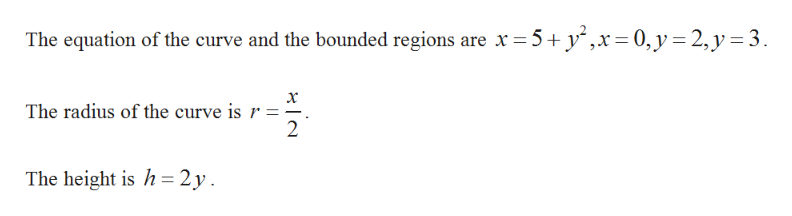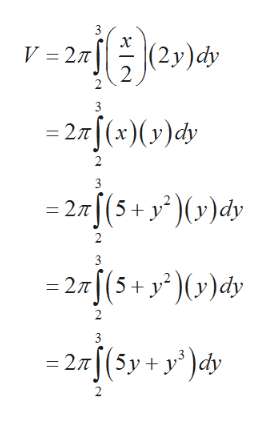# Use the method of cylindrical shells to find the volume V of the solid obtained by rotating the region bounded by the given curves about the x-axis.x = 5 + y2, x = 0, y = 2, y = 3

Question
14 views

Use the method of cylindrical shells to find the volume V of the solid obtained by rotating the region bounded by the given curves about the x-axis.
x = 5 + y2, x = 0, y = 2, y = 3

check_circle

Step 1help_outlineImage TranscriptioncloseThe equation of the curve and the bounded regions are x5+ y,x= 0,y 2, y 3 x The radius of the curve is r =- 2 The height is h 2y fullscreen
Step 2

Now calculate the volume of the solid by...help_outlineImage Transcriptionclose3 V 27T (2y)dy 3 - 27(x)(y)dy 2 3 - 27(5+y')(y)dy 2 3 - 2(5+ y2)y)dy 2 3 - 2T(5y+ y)dy fullscreen

### Want to see the full answer?

See Solution

#### Want to see this answer and more?

Solutions are written by subject experts who are available 24/7. Questions are typically answered within 1 hour.*

See Solution
*Response times may vary by subject and question.
Tagged in

### Calculus